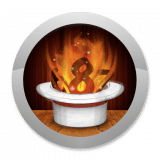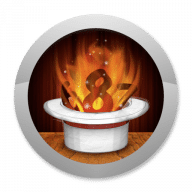Mathemagics
1.1

0.0

Mathemagics# Mathemagics1.1

27 December 2013

Learn to do arithmetic quickly and easily.

## Overview

With Mathemagics, learn how to add, subtract, multiply, divide, and square at lightning fast speed.
• Learn lessons at your pace
• Learn how to mentally check your calculations
• Perfect for students and adults alike
• Practice each lesson and progress to the level of Mathemagician
• Play games with your choice of lessons or a random mix
• Stats tracking for each game
• Full screen mode
Learn and practice the tricks of mental math calculation in a fun and engaging application. Study any of the math tricks and then practice them as you progress through various levels of proficiency. Tease your brain with a quick practice session while waiting in lines, riding in the car, or on the plane. Only the basic math skills of addition, subtraction and simple multiplication and division are needed to achieve astonishing results.

Students: Baffle your math teachers by providing answers faster than thought possible.
Teachers: Inspire your students as you pull answers to math problems out of thin air.

Master these math tricks:

• Multiply as high as 20x20
• Square a number ending in 5
• Square any two digit number
• Doubling and halving
• Multiply any number by 4
• Multiply any number by 5
• Multiply any number by 9
• Multiply any number by 25
• Multiply any number by 99
• Squares from 41 to 59
• Round number multiplication
• Divide any number by 5
• Multiply by 11
• Multiply in the 90's
• Square numbers in the 50's
• Multiply differing by 2,4,6
• Multiply if 1's sum is 10
• Multiply two close numbers
• Sum of first n even numbers
• Sum of first n odd numbers
• Nines complement
• Tens complement
• Large number subtraction
• Evenly divisible by 3
• Evenly divisible by 4
• Evenly divisible by 5
• Evenly divisible by 6
• Evenly divisible by 7
• Evenly divisible by 8
• Evenly divisible by 9
• Evenly divisible by 10
• Evenly divisible by 11
• Evenly divisible by 12
• Square numbers in the 20's
• Square numbers in the 30's
• Square numbers in the 60's
• Square numbers in the 70's
• Square numbers in the 80's
• Square numbers in the 100's
• Square numbers in the 200's
• Square numbers in the 300's
• Square numbers in the 400's
• Square numbers in the 500's
• Square numbers in the 700's
• Square numbers in the 800's
• Square numbers in the 900's
• Casting Out Nines Digit Sum
• Check Addition by Casting Nines
• Check Subtraction by Casting Nines
• Check Multiplication by Casting Nines
• Check Division by Casting Nines
• Casting Out Elevens Digit Sum
• Check Addition by Casting Elevens
• Check Subtraction by Casting Elevens
• Check Multiplication by Casting Elevens
• Check Division by Casting Elevens
Prepare for the math section on the SAT, PSAT, ACT, GRE, GMAT, MCAT, or any other standardized test. Don't waste time with a calculator on the few tests that allow them. Use Mathemagics as a tutor to quicken up your test taking abilities.

Perfect training for the UIL Mathematics and Number Sense Contests.

Activate and challenge your brain to expand its ability to think efficiently and have fun at the same time. Train yourself to break problems down in to smaller more manageable pieces in order to reach solutions quicker and more accurately than anyone else.

## What's new in Mathemagics

Version 1.1:
• Compatibility update
• Bug fixes

#### Join over 500,000 subscribers.

Subscribe for our newsletter with best Mac offers from MacUpdate.

How would you rate Mathemagics app?